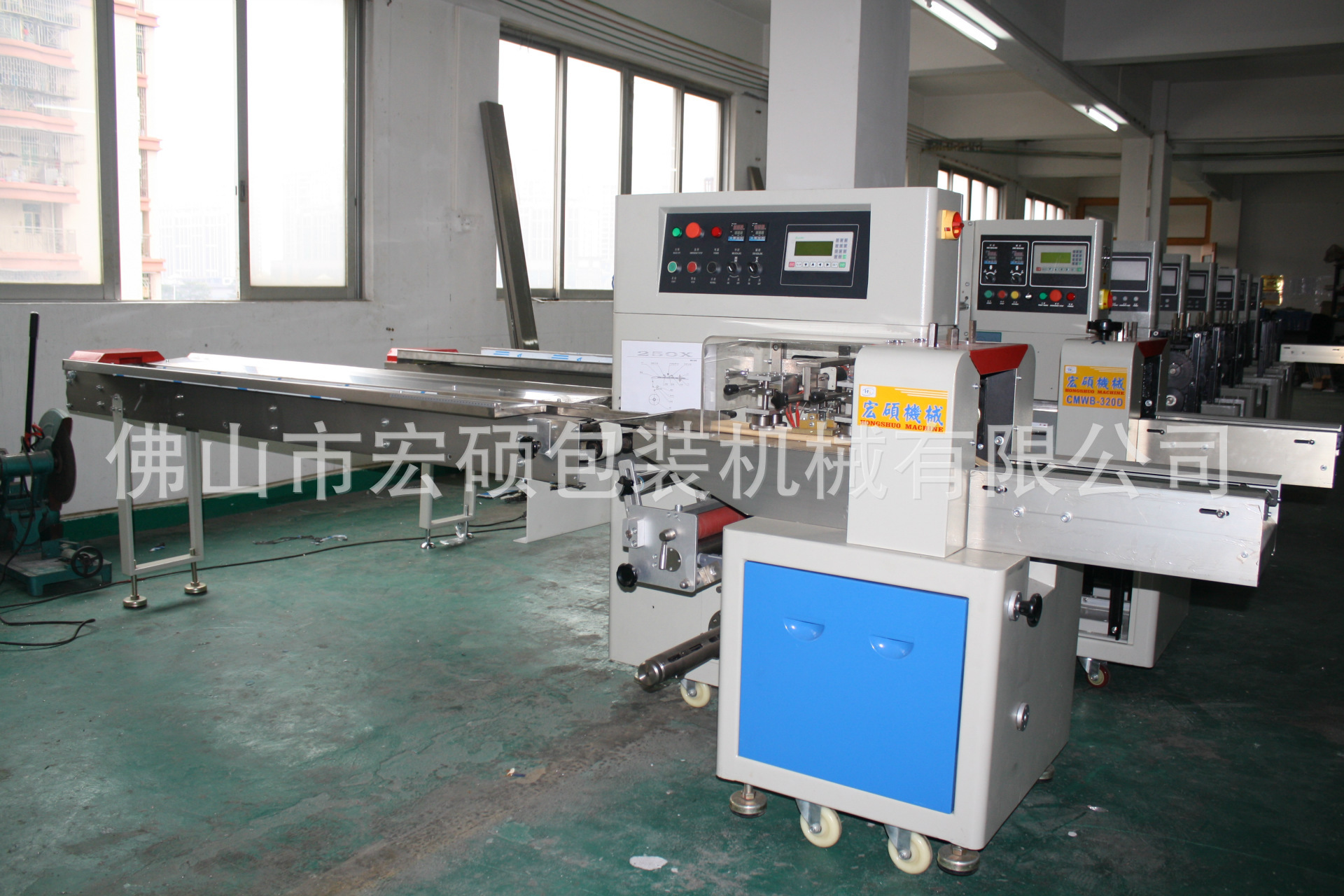# 自动包装机械,铰链包装机械,铰链机械,下走纸机械,

## 距离截止时间还剩：长期采购

• 需求数量：500
• 包装需求：
• 所在地：广东
• 已有847人浏览• 暂无相关资料！

### 产品详细说明

r 佛山市宏硕包装机械有限公司位于禅城区镇安大道十字冲星星工业二区B座。是一家高科技软硬开发生产企业作为专业定位于机械制造业、机械加工业、自动化生产线等机械研究及开发型的企业，始终遵循以人为本，以产品开发为中心，以价值管理为手段，实现社会价值最大化的企业管理理念r

r

r 本公司除经营枕式、立式、自动配套系列食品包装机械、还提供各类机械零配件加工.　　本公司产品远销国内外并深得广大用户信赖，产品三包、代办托运、详细资料电话或电邮索取即寄。热忱欢迎贵方选购使用本公司产品。r

rr r r r r r
r r r r r r r r
 r r HS-250X 下走纸枕式包装机rr(一)主要性能和结构特点r1.双变频器控制，袋长即设即切，无需调节空走，一步到位，省时省膜。r2.人机界面，参数设定方便快捷。r3.故障自诊断功能，故障显示一目了然。r4.高感度光电眼色标跟踪，数字化输入封切位置，使封切位置更加准确。r5.温度独立PID控制，更好适合各种包装材质。r6.定位停机功能，不粘刀，不浪费包膜。r7.传动系统简洁，工作更可靠，维护保养更方便。r8.所有控制由软件实现，方便功能调整和技术升级，永不落后。 r r
r r

r  r

r
r

r  r

rr r r r r r
r r r r r r r r
 r r r r(r r二)适用范围r r r铰链 导轨 合页 拉手 轴承 线圈  铁盒 钢管等五金产品进行包装。rrrrrrr r
r r

rr
r
r

r
r

r  r

rr r r r r r
r r r r r r r r
 r r r(三)选配装置r1.打码机r2.直纹中封轮r3.网纹端封模r4.超大触摸屏 r r
r r

r
r

r
r

r
r

rr r r r r r
r r r r r r r r
r

r
r(
)技术规格 r

r r r r r r r r r r r r r r r r r r r r r r r r r r r r r r r r r r r r r r r r r r r r r r r r
 r r 机型 r r r r HS-250Xr r r r 薄膜宽度 r r r r Max.250mmr r r r 制袋长度r r r r 65～190mm或120~280mmr r r r 制袋宽度r r r r 30～110mmr r r r 产品高度r r r r Max.40mmr r r r 膜卷直径r r r r Max.320mmr r r r 包装速度r r r r 40～230包/分r r r r 电源规格r r r r 220V,50/60HZ,2.4KVAr r r r 机器尺寸r r r r (L)3920×(W)670×(H)1320r r r r 机器质量r r r r 800Kgr r r r 备   注r r r r  r r
r

r
r

r
r r

r
r

r

500 价格面议 广东

### 相关询价单推荐

• #### 食品包装机械,宏硕包

采购数量500价格需求：

包装需求：

来自[全国]的采购商

已有975人浏览

立即报价

有效期至：长期有效

• #### 自动包装机械,铰链包

采购数量500价格需求：

包装需求：

来自[全国]的采购商

已有847人浏览

立即报价

有效期至：长期有效

• #### 自动包装机械,物流机

采购数量5000价格需求：

包装需求：

来自[全国]的采购商

已有577人浏览

立即报价

有效期至：长期有效

• #### 自动包装机械,专业包

采购数量1000价格需求：

包装需求：

来自[全国]的采购商

已有607人浏览

立即报价

有效期至：长期有效

• #### 包装机械,湿巾包装机

采购数量500价格需求：

包装需求：

来自[全国]的采购商

已有531人浏览

立即报价

有效期至：长期有效

• #### 包装机械,机械,全国机

采购数量500价格需求：

包装需求：

来自[全国]的采购商

已有756人浏览

立即报价

有效期至：长期有效

• #### 包装机械,封口机械,包

采购数量100价格需求：

包装需求：

来自[全国]的采购商

已有761人浏览

立即报价

有效期至：长期有效